##### GED Test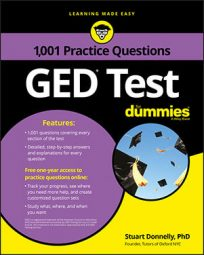Radical problems on the GED Math test usually involve simplifying and reducing terms to find the answer. These questions are easy to recognize because they always include a radical symbol: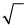## Practice Questions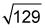is between which numbers?

A. 10 and 11 B. 11 and 12 C. 14 and 15 D. 64 and 65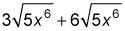completely.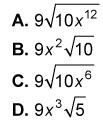1. The correct answer is B.

129 lies between the square numbers 121 and 144. Therefore,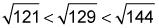which reduces to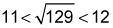Hence, Choice (B) is the correct answer.

2. The correct answer is D.

When combining radicals, you first have to check that the radicands (the expressions under the radical symbols) are the same. Because they are in this problem, you add the two coefficients together and leave the radicands alone: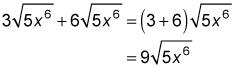Because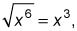you can simplify the expression even further to give you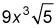Hence, Choice (D) is correct.